# Building the diffusion operator

Part of the quantum computing series
Part 1: Quantum Computing in a Nutshell
Part 2: More on quantum gates
Part 3: Deutch-Josza Algorithm
Part 4: Grover’s algorithm
Part 5: Building the quantum oracle

Let’s more precisely define the Grover diffusion operator D we used for Grover’s algorithm, and see why it functions to flip amplitudes over the average amplitude.

First off, here’s a useful bit of shorthand we’ll use throughout the post. We define the uniform superposition over states as |s⟩: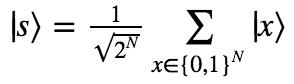We previously wrote that flipping an amplitude ax over the average of all amplitudes ā involved the transformation ax → 2ā – ax. This can be understood by a simple geometric argument: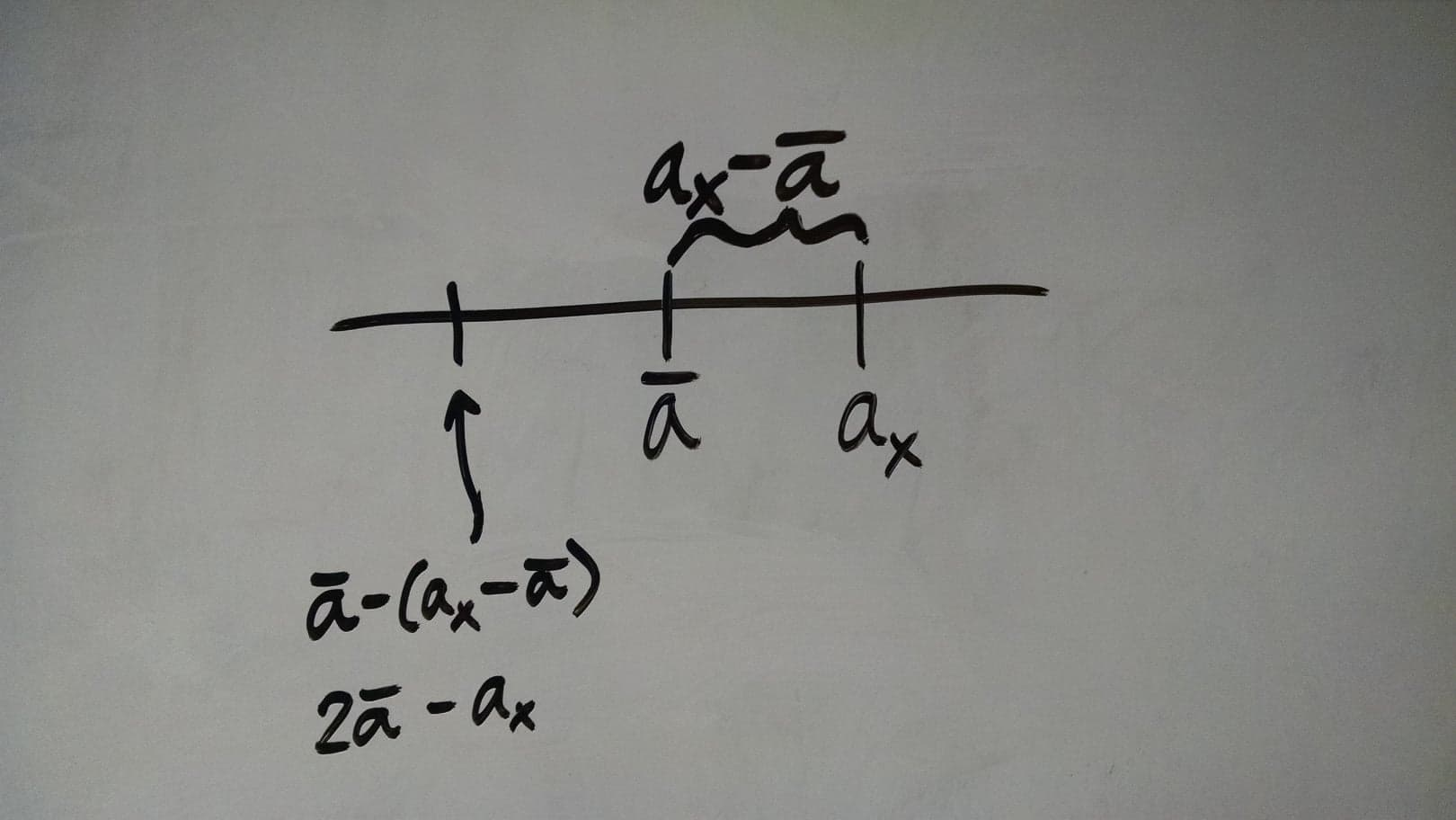Now, the primary challenge is to figure out how to build a quantum gate that returns the average amplitude of a state. In other words, we want to find an operator A such that acting on a state ⟩ gives:If we can find this operator, then we can just define D as follows: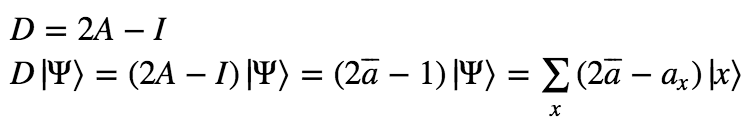It turns out that we can define A solely in terms of the uniform superposition.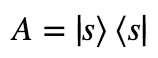As a matrix, A would look like:Proof that this satisfies the definition: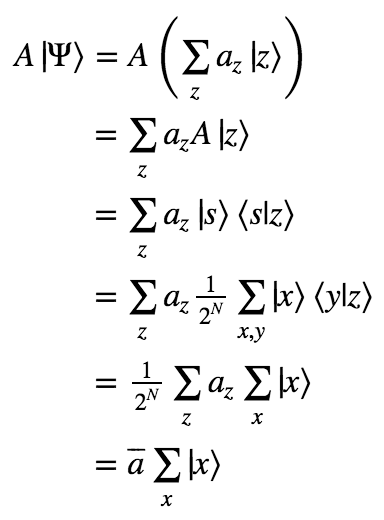Thus we have our full definition of D!As a matrix, D looks like: